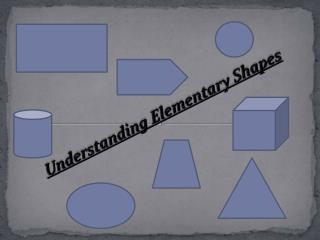DownloadDownload PresentationUnderstanding Elementary Shapes

# Understanding Elementary Shapes

Download Presentation## Understanding Elementary Shapes

- - - - - - - - - - - - - - - - - - - - - - - - - - - E N D - - - - - - - - - - - - - - - - - - - - - - - - - - -
##### Presentation Transcript

1. Understanding Elementary Shapes

2. Introduction All the shapes we see around us are formed using curves or lines. We can see corners, edges, planes, open curves and closed curves in our surroundings. We organise them into line segments, angles, triangles, polygons and circles.

3. Angles – ‘ Right’ and ‘Straight’ We know that China is to the north of India. Sri Lanka is to the south. We also know that sun rises in the east and sets in the west. There are four main directions they are North(N), South(S), East(E) and West(W). N N E N N E E W S S

4. The turn from north to east is by a right angle. The turn from north to south is by two right angles, it is called a straight angle (NS is a straight line) Complete Angle Turning by two straight angles(or four right angles) in the same direction makes a full turn. This one complete turn is called one revolution. The angle for one revolution is a complete angle. We can see such revolutions on clock- faces. Suppose the hand of a clock starts at 12 and goes round until it reaches at 12 again. So it has completed one revolution. Acute Angle An angle smaller than a right angle is called an acute angle.

5. Obtuse Angle If an angle is larger than a right angle, but less than a straight angle, it is called an obtuse angle Book reading Desk

6. Naming Triangles Based on Sides • Scalene Triangle • A triangle having all three unequal sides is called a scalene triangle. • Isosceles Triangle • A triangle having two equal sides is called an isosceles triangle. • Equilateral Triangle • A triangle having three equal sides is called an equilateral triangle. Scalene Triangle Equilateral Triangle Isosceles Triangle

7. Naming triangles based on angles • Acute angled triangle • If each angle is less than 900, then the triangle is called an acute angled triangle. • Right Angled Triangle • If any one angle is a right angle, then the triangle is called a right angled triangle.Obtuse Angled Triangle • If any one angle is grater than 900, then the triangle is called an obtuse angled triangle. Obtuse Triangle Acute Triangle Right Triangle

8. Polygons Polygon is a closed figure made up of line segments

9. Hexagon Quadrilateral Pentagon Triangle Octagon Heptagon

10. Three Dimensional Shapes Cuboid Cube Cylinder Sphere

11. Faces, Edges and Vertices Consider a cube. Each side of the cube is a flat surface called a flat face. Two faces meet at a line segment called an edge. Three edges meet at a point called vertex. Face Edge Vertex

12. Match the following: (i)Straight angle (a) less than one fourth of a revolution. (ii) Right angle (b) More than half a revolution. (iii) Acute angle (c) half of a revolution. (iv)Obtuse angle (d) One fourth of a revolution. (v)reflex angle (e) between ¼ and ½ of a revolution. Say true or false (a) The measure of an acute angle <900. (b) The measure of an obtuse angle <900. (c)The measure of a reflex angle > 1800 . (d) The measure of one complete revolution= 3600. (e) If, m<A = 530 and m<B= 350, then measure of <A > measure of <B.